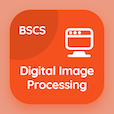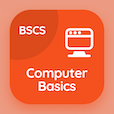Computer Science Courses Online

Digital Image Processing Exam Prep

Digital Image Processing Practice Test 41

# Introduction to Wavelet and Multiresolution Processing MCQ Questions PDF Download - 41

The Book Introduction to Wavelet and Multiresolution Processing Multiple Choice Questions and Answers (MCQs), introduction to wavelet and multiresolution processing quiz answers PDF chapter 10-41 to study online courses, digital image processing tests. Study Wavelet and Multiresolution Processing MCQ trivia questions, introduction to wavelet and multiresolution processing Multiple Choice Questions (MCQ Quiz) for online college degrees. The eBook Introduction to Wavelet and Multiresolution Processing MCQs App Download: introduction to wavelet and multiresolution processing, wavelet and multiresolution processing basics, line detection in image segmentation, 10d discrete fourier transform, fundamentals of image segmentation test prep for cheapest online computer science degree.

The MCQ: K multiplication constants in digital filters are called PDF, "Introduction to Wavelet and Multiresolution Processing" App Android & iOS (Free) with multipliers, co efficient, subtractors, and filter coefficients choices for computer and information science. Practice wavelet and multiresolution processing questions and answers, Google eBook to download free sample for top online computer science programs.

## Image Processing: Introduction to Wavelet & Multiresolution Processing MCQ Quiz

MCQ: K multiplication constants in digital filters are called

A) co efficient
B) multipliers
C) subtractors
D) filter coefficients

MCQ: The size of the base image will be

A) N-1 x N-1
B) N+1 x N-1
C) N-1 x N
D) N x N

MCQ: Vertical lines are angles at

A) 0
B) 30
C) 45
D) 90

MCQ: Odd functions are said to be

A) symmetric
B) antisymmetric
C) periodic
D) aperiodic

MCQ: If R is the entire region of the image then union of all segmented parts should be equal to

A) R
B) R'
C) Ri
D) Rn

### Mock Tests: Digital Image Processing Course Prep

Download Digital Image Processing Quiz App, Computer Basics MCQs App and Database Management System MCQ App for Android & iOS devices. These Apps include complete analytics of real time attempts with interactive assessments. Download Play Store & App Store Apps & Enjoy 100% functionality with subscriptions!